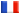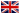# Séminaire de Cryptographie

## Alain Passelègue### An Algebraic Framework for Pseudorandom Functions and Applications to Related-Key Security

Pseudorandom functions (PRFs) are one of the most fundamental primitives in cryptography. In this work, we provide a new algebraic framework which encompasses many of the existing algebraic PRFs, including the ones by Naor and Reingold (FOCS'97), by Lewko and Waters (CCS'09), and by Boneh, Montgomery, and Raghunathan (CCS'10), as well as the related-key-secure PRFs by Bellare and Cash (Crypto'10) and by Abdalla \etal (Crypto'14). To achieve this goal, we introduce two versions of our framework. The first, termed linearly independent polynomial security, states that the values $(g^{P_1(\vec{a})}, \ldots, g^{P_q(\vec{a})})$ are indistinguishable from a random tuple of the same size, when $P_1, \ldots, P_q$ are linearly independent multivariate polynomials of the secret key vector $\vec{a}$. The second, which is a natural generalization of the first framework, additionally deals with constructions based on the decision linear and matrix Diffie-Hellman assumptions. In addition to unifying and simplifying proofs for existing schemes, our new framework also yields several new results, such as related-key security with respect to arbitrary permutations of polynomials. All our constructions are in the standard model and do not require the existence of multilinear maps.